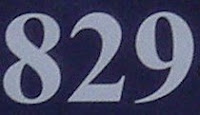## Tuesday, September 14, 2010

### 829

829 is a prime number.

829 has a representation as a sum of two squares: 829 = 102 + 272.

827 and 829 form a twin prime pair.

829 is the hypotenuse of a primitive Pythagorean triple: 8292 = 5402 + 6292.

829 is a divisor of 59 - 1.

829 is the sum of three consecutive primes: 829 = 271 + 277 + 281.829 is a telephone area code for the Dominican Republic.

Source: WolframAlpha